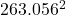## Anakin Skywalker’s pod racer has a mass of 450 kg. If the top speed of this racer is 947 km/h, what is the maximum kinetic energy it ca

Question

Anakin Skywalker’s pod racer has a mass of 450 kg. If the top speed of this racer is 947
km/h, what is the maximum kinetic energy it can have​

in progress 0
2 months 2021-07-21T09:54:27+00:00 2 Answers 0 views 0

15,569,653.3 Joules(J will be your sum

15,569,653.3 Joules(J)

Explanation:

The equation used to find Kinetic Energy (KE) is

KE =mYou have been given

m = 450kg

v = 947km/h

KE = ???

Firstly, we need to convert the km/h into m/s as this is the unit used in the KE equation

This can be done by dividing by 3.6

947km/h = 263.056m/s

Substitute you values into the equation

KE =mKE =* 450 *KE = 15,569,653.3 Joules(J)